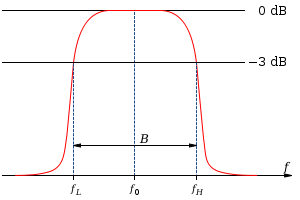xCenter frequencyEncyclopediaIn electrical engineering
Electrical engineering
Electrical engineering is a field of engineering that generally deals with the study and application of electricity, electronics and electromagnetism. The field first became an identifiable occupation in the late nineteenth century after commercialization of the electric telegraph and electrical...

and telecommunications, the center frequency of a filter or channel is a measure of a central frequency between the upper and lower cutoff frequencies
Cutoff frequency
In physics and electrical engineering, a cutoff frequency, corner frequency, or break frequency is a boundary in a system's frequency response at which energy flowing through the system begins to be reduced rather than passing through.Typically in electronic systems such as filters and...

. It is usually defined as either the arithmetic mean
Arithmetic mean
In mathematics and statistics, the arithmetic mean, often referred to as simply the mean or average when the context is clear, is a method to derive the central tendency of a sample space...

or the geometric mean
Geometric mean
The geometric mean, in mathematics, is a type of mean or average, which indicates the central tendency or typical value of a set of numbers. It is similar to the arithmetic mean, except that the numbers are multiplied and then the nth root of the resulting product is taken.For instance, the...

of the lower cutoff frequency and the upper cutoff frequency of a band-pass system
Band-pass filter
A band-pass filter is a device that passes frequencies within a certain range and rejects frequencies outside that range.Optical band-pass filters are of common usage....

or a band-stop system
Band-stop filter
In signal processing, a band-stop filter or band-rejection filter is a filter that passes most frequencies unaltered, but attenuates those in a specific range to very low levels. It is the opposite of a band-pass filter...

.

Typically, the geometric mean is used in systems based on certain transformations of lowpass filter designs, where the frequency response
Frequency response
Frequency response is the quantitative measure of the output spectrum of a system or device in response to a stimulus, and is used to characterize the dynamics of the system. It is a measure of magnitude and phase of the output as a function of frequency, in comparison to the input...

is constructed to be symmetric on a logarithmic
Logarithmic scale
A logarithmic scale is a scale of measurement using the logarithm of a physical quantity instead of the quantity itself.A simple example is a chart whose vertical axis increments are labeled 1, 10, 100, 1000, instead of 1, 2, 3, 4...

frequency scale. The geometric center frequency corresponds to a mapping of the DC response of the prototype lowpass filter, which is a resonant frequency sometimes equal to the peak frequency of such systems, for example as in a Butterworth filter
Butterworth filter
The Butterworth filter is a type of signal processing filter designed to have as flat a frequency response as possible in the passband so that it is also termed a maximally flat magnitude filter...

.

The arithmetic definition is used in more general situations, such as in describing passband
Passband
A passband is the range of frequencies or wavelengths that can pass through a filter without being attenuated.A bandpass filtered signal , is known as a bandpass signal, as opposed to a baseband signal....

telecommunication
Telecommunication
Telecommunication is the transmission of information over significant distances to communicate. In earlier times, telecommunications involved the use of visual signals, such as beacons, smoke signals, semaphore telegraphs, signal flags, and optical heliographs, or audio messages via coded...

systems, where filters are not necessarily symmetric but are treated on a linear frequency scale for applications such as frequency-division multiplexing
Frequency-division multiplexing
Frequency-division multiplexing is a form of signal multiplexing which involves assigning non-overlapping frequency ranges to different signals or to each "user" of a medium.- Telephone :...

.